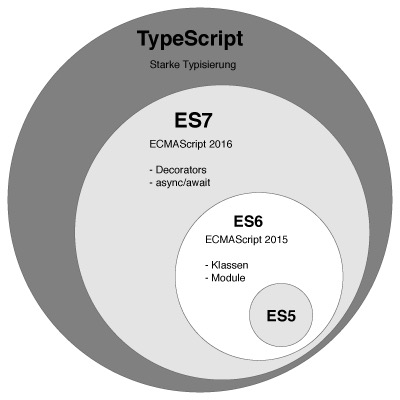Time FlyingTypeScript 并不是一个完全新的语言, 它是 JavaScript 的超集，为 JavaScript 的生态增加了类型机制，并最终将代码编译为纯粹的 JavaScript 代码。

## TypeScript 简介

TypeScript 由 Microsoft(算上 Angular 2 的话加上 Google)开发和维护的一种开源编程语言。 它支持 JavaScript 的所有语法和语义，同时通过作为 ECMAScript 的超集来提供一些额外的功能，如类型检测和更丰富的语法。下图显示了 TypeScript 与 ES5，ES2015，ES2016 之间的关系。## 使用 TypeScript 的原因

JavaScript 是一门弱类型语言，变量的数据类型具有动态性，只有执行时才能确定变量的类型，这种后知后觉的认错方法会让开发者成为调试大师，但无益于编程能力的提升，还会降低开发效率。TypeScript 的类型机制可以有效杜绝由变量类型引起的误用问题，而且开发者可以控制对类型的监控程度，是严格限制变量类型还是宽松限制变量类型，都取决于开发者的开发需求。添加类型机制之后，副作用主要有两个：增大了开发人员的学习曲线，增加了设定类型的开发时间。总体而言，这些付出相对于代码的健壮性和可维护性，都是值得的。

## 数据类型

### String 类型

``````let name: string = 'muyy'
let name2: String = 'muyy'``````

### Boolen 类型

boolean 是 true 或 false 的值，所以 `let isBool3: boolean = new Boolean(1)` 就会编译报错，因为 new Boolean(1) 生成的是一个 Bool 对象。

``let isBool1: boolean = false``

### Number 类型

``let number: number = 10;``

### Array 类型

``````let arr:number[] = [1, 2, 3, 4, 5];
let arr2:Array<number> = [1, 2, 3, 4, 5];

let arr3:string[] = ["1","2"];
let arr4:Array<string> = ["1","2"];``````

### Tuple 类型

Tuple 类型相对于 Array 类型, 其允许元素的类型不一定相同。

``````let x: [string, number]
x = ['a', 1]``````

### Enums 类型

``````enum Role {Employee = 3, Manager, Admin}
let role: Role = Role.Employee
console.log(role) // 3
console.log(Role) // Manager``````

### Any 类型

any 是默认的类型，其类型的变量允许任何类型的值：

``````let notSure:any = 10;
let notSure2:any[] = [1,"2",false];``````

### Void 类型

JavaScript 没有空值 Void 的概念，在 TypeScirpt 中，可以用 void 表示没有任何返回值的函数：

``````function alertName(): void {
console.log('My name is muyy')
}``````

### Unknow 类型

new-unknown-top-type, 比 any 更加安全的类型

### Never 类型

``````// Function returning never must have unreachable end point
function error(message: string): never {
throw new Error(message);
}

// Inferred return type is never
function fail() {
return error("Something failed");
}

// Function returning never must have unreachable end point
function infiniteLoop(): never {
while (true) {
}
}``````

## 函数

### 为函数定义类型

``````function add(x: string, y: string): string{
return "Hello TypeScript";
}

let add2 = function(x: string, y: string): string{
return "Hello TypeScript";
}

let add3: (x: string, y: string) => string = function(x: string, y: string): string{
return "Hello TypeScript";
}``````

### 可选参数和默认参数

JavaScript 里，每个参数都是可选的，可传可不传。 没传参的时候，它的值就是 undefined 。 在 TypeScript 里我们可以在参数名旁使用?实现可选参数的功能。 比如，我们想让 lastname 是可选的:

``````function buildName(firstName: string, lastname?: string){
console.log(lastname ? firstName + "" + lastname : firstName)
}

let res1 = buildName("鸣","人"); // 鸣人
let res2 = buildName("鸣"); // 鸣
let res3 = buildName("鸣", "人", "君"); // Supplied parameters do not match any signature of call target.``````

``````function buildName2(firstName = "鸣", lastName?: string){
console.log(firstName + "" + lastName)
}

let res4 = buildName2("人"); // undefined人
let res5 = buildName2(undefined, "人"); // 鸣人``````

## 类

### 类

``````class Person{
name:string; // 这个是对后文this.name类型的定义
age:number;
constructor(name:string,age:number){
this.name = name;
this.age = age;
}
print(){
return this.name + this.age;
}
}

let person:Person = new Person('muyy',23)
console.log(person.print()) // muyy23``````

### 继承

``````class Person{
public name:string;  // public、private、static 是 typescript 中的类访问修饰符
age:number;
constructor(name:string,age:number){
this.name = name;
this.age = age;
}
tell(){
console.log(this.name + this.age);
}
}

class Student extends Person{
gender:string;
constructor(gender:string){
super("muyy",23);
this.gender = gender;
}
tell(){
console.log(this.name + this.age + this.gender);
}
}

var student = new Student("male");
student.tell();  // muyy23male``````

### 存储器

TypeScript 支持通过 getters/setters 来截取对对象成员的访问。 它能帮助你有效的控制对对象成员的访问。

``````class Hello{
private _name: string;
private _age: number;
get name(): string {
return this._name;
}
set name(value: string) {
this._name = value;
}
get age(): number{
return this._age;
}
set age(age: number) {
if(age>0 && age<100){
console.log("年龄在0-100之间"); // 年龄在0-100之间
return;
}
this._age = age;
}
}

let hello = new Hello();
hello.name = "muyy";
hello.age = 23
console.log(hello.name); // muyy``````

## 接口

### 接口

TypeScript 的核心原则之一是对值所具有的结构进行类型检查。在 TypeScript 里，接口的作用就是为这些类型命名和为你的代码或第三方代码定义契约。

``````interface LabelValue{
label: string;
}

function printLabel(labelObj: LabelValue){
console.log(labelObj.label);
}

let myObj = {
"label":"hello Interface"
};
printLabel(myObj);``````

LabelledValue 接口就好比一个名字，它代表了有一个 label 属性且类型为 string 的对象。只要传入的对象满足上述必要条件，那么它就是被允许的。

### 可选属性

``````interface Person{
name?:string;
age?:number;
}

function printInfo(info:Person){
console.log(info);
}

let info = {
"name":"muyy",
"age":23
};

printInfo(info); // {"name": "muyy", "age": 23}

let info2 = {
"name":"muyy"
};

printInfo(info2); // {"name": "muyy"}``````

### 函数类型

``````interface SearchFunc{
(source: string, subString: string): boolean;
}

let mySearch: SearchFunc;
mySearch = function(source: string,subString: string){
return source.search(subString) !== -1;
};

console.log(mySearch("鸣人","鸣")); // true
console.log(mySearch("鸣人","缨")); // false``````

### 可索引类型

``````interface StringArray{
[index: number]: string;
}

let MyArray: StringArray;
MyArray = ["是","云","随","风"];
console.log(MyArray); // 随``````

### 类类型

``````interface ClockInterface{
currentTime: Date;
setTime(d: Date);
}

class Clock implements ClockInterface{
currentTime: Date;
setTime(d: Date){
this.currentTime = d;
}
constructor(h: number, m: number) {}
}``````

### 继承接口

``````interface Shape{
color: string;
}

interface PenStroke{
penWidth: number;
}

interface Square extends Shape,PenStroke{
sideLength: number;
}

let s = <Square>{};
s.color = "blue";
s.penWidth = 100;
s.sideLength = 10;``````

## 模块

TypeScript 与 ECMAScript 2015 一样，任何包含顶级 import 或者 export 的文件都被当成一个模块。

``````export interface StringValidator{
isAcceptable(s:string): boolean;
}

var strReg = /^[A-Za-z]+\$/;
var numReg = /^[0-9]+\$/;

export class letterValidator implements StringValidator{
isAcceptable(s:string): boolean{
return strReg.test(s);
}
}

export class zipCode implements StringValidator{
isAcceptable(s: string): boolean{
return s.length == 5 && numReg.test(s);
}
}``````

## 泛型

### 初探泛型

``````function Hello<T>(arg:T):T{
return arg;
}

let outPut = Hello<string>('Hello Generic');
let output2 = Hello('Hello Generic')

console.log(outPut);
console.log(outPut2);``````